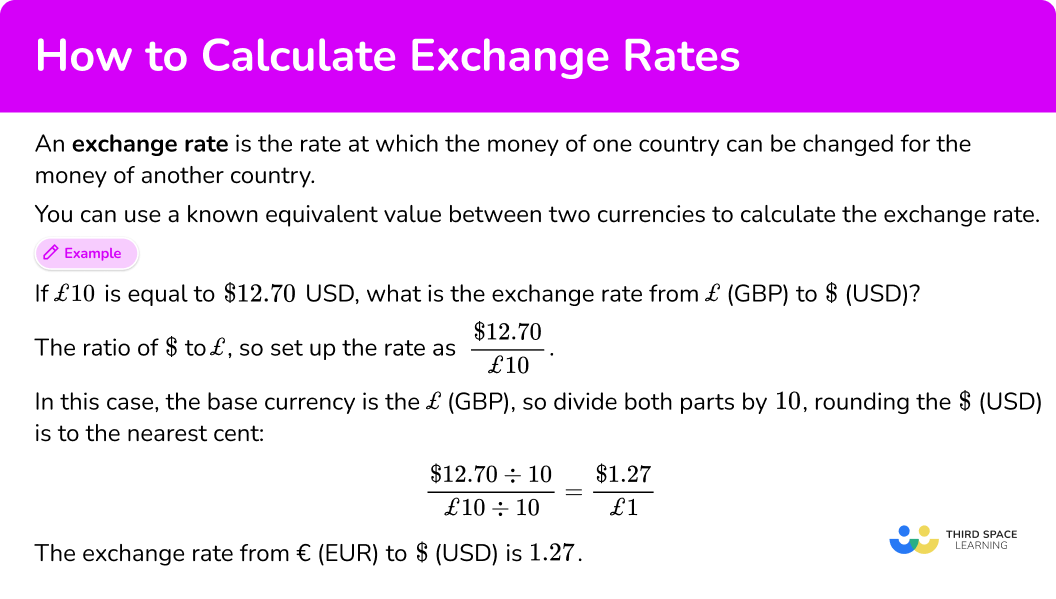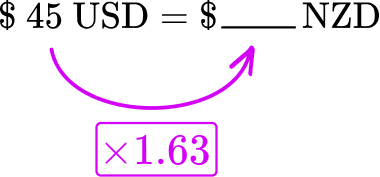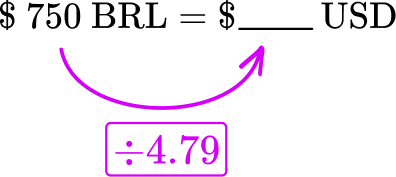Math resources Ratio and proportion Ratio

How to calculate exchange rates

# How to calculate exchange rates

Here you will learn about how to calculate exchange rates, including how to convert between American dollars and foreign currencies.

Students will first learn how to calculate exchange rates as part of ratios and proportions in 6 th grade and 7 th grade.

## What is an exchange rate?

An exchange rate is the rate at which the money of one country can be changed for the money of another country. It can also be referred to as a foreign exchange rate and be seen as the price of one currency expressed in terms of another currency.

In the United States, our currency is the American dollar. The exchange rate tells us the value of \$1 in terms of a foreign country’s currency. Different countries have different currencies. For example, Some major currencies are… You can use a known equivalent value between two currencies to calculate the exchange rate. For example, If £10 is equal to \$12.70 \; USD, what is the exchange rate from £ \; (GBP) to \$\; (USD)? When calculating the currency exchange rate from £ \; (GBP) to \$ \; (US dollars ), you want to know how many \$are equal to £1. This is the ratio of \$ to £, so set up the rate as \cfrac{\$12.70}{£ 10}. In this case, the base currency is the £ \; (GBP), so divide both parts by 10, rounding the \$ \; (USD) to the nearest cent:

\cfrac{\$12.70 \div 10}{£ 10 \div 10}=\cfrac{\$ 1.27}{£ 1}

The exchange rate from £ \; (GBP) to \$\; (USD) is 1.27. The exchange rates (currency conversions) are constantly changing depending on the country’s economic status. It is the role of the central bank to monitor these fluctuations and adjust the country’s monetary policy accordingly, which in turn impacts the currency’s value in the forex trading market (foreign exchange market). Using a currency’s exchange rate, you can convert between currencies. To do this, you need to either multiply or divide the quantity you are trying to convert with the exchange rate. To convert from US dollars (USD) to Mexican pesos (MXN) , you must multiply by the exchange rate. For example, So \$15 \; USD would be \$251.40 \; MXN because \$ 15 \; U S D \times 16.76=\$251.4 \; M X N. For example, The exchange rate from \$ \; (USD) to £ \; (GBP) is 1.28.

To convert a British pound (GBP) into US dollars (USD) , you must divide by the exchange rate.

So, £40 would be \$31.25 because £ 40 \; G B P \div 1.28=\$ 31.25 \; U S D

### What is an exchange rate?## Common Core State Standards

How does this relate to 6 th grade math and 7 th grade math?

• Grade 6 – Ratios and Proportional Relationships (6.RP.A.3b)
Solve unit rate problems including those involving unit pricing and constant speed. For example, if it took 7 hours to mow 4 lawns, then at that rate, how many lawns could be mowed in 35 hours? At what rate were lawns being mowed?

• Grade 7 – Ratios and Proportional Relationships (7.RP.A.1)
Compute unit rates associated with ratios of fractions, including ratios of lengths, areas and other quantities measured in like or different units. For example, if a person walks \cfrac{1}{2} mile in each \cfrac{1}{4} hour, compute the unit rate as the complex fraction \cfrac{\cfrac{1}{2}}{\cfrac{1}{4}} miles per hour, equivalently 2 miles per hour.

## How to calculate exchange rates

In order to calculate exchange rates:

1. Use the information given to set up a rate.
2. Divide both parts by the base currency.
3. State the final exchange rate with the correct currency symbols.

## How to calculate exchange rates examples

### Example 1: converting from EUR / USD

€9 is equal to \$9.87 \; USD. What is the exchange rate from € \; (EUR) to \$ \; (USD)?

1. Use the information given to set up a rate.

When calculating the currency exchange rate from € \; (EUR) to \$\; (USD or US dollars ), you want to know how many \$ are equal to €1. This is the ratio of \$to €, so set up the rate as \cfrac{\$ 9.87}{€ 9}.

2Divide both parts by the base currency.

In this case, the base currency is the euro \, € \; (EUR), so divide both parts by 9, rounding the \$\; (USD) to the nearest cent: \cfrac{\$ 9.87 \div 9}{€ 9 \div 9}=\cfrac{\$1.10}{€ 1} 3State the final exchange rate with the correct currency symbols. The exchange rate from € \; (EUR) to \$ \; (USD) is 1.10.

### Example 2: converting from AUD / USD

\$9.09 \; AUD is equal to \$6 \; USD. What is the exchange rate from \$\; (USD) to \$ \; (AUD)?

When calculating the currency exchange rate from \$\; (USD) to \$ \; (AUD), you want to know how many \$\; (AUD) are equal to \$1 \; (USD).

This is the ratio of \$\; (AUD) to \$ \; (USD) so set up the rate as \cfrac{\$9.09 \text { AUD }}{\$ 6 \text{ USD }}.

In this case, the base currency is \$\; (USD), so divide both parts by 6, rounding the \$ \; (AUD) to the nearest cent:

\cfrac{\$9.09 \, A U D \div 6}{\$ 6 \, U S D \div 6}=\cfrac{\$1.52 \, A U D}{\$ 1 \, U S D}

The exchange rate from \$\; (USD) to \$ \; (AUD) is 1.52.

### Example 3: converting from JPY / USD

¥26 \; JPY is equal to \$0.18 \; USD. What is the exchange rate from \$ \; (USD) to ¥ \; (JPY)?

When calculating the currency exchange rate from \$\; (USD) to ¥ \; (JPY), you want to know how many ¥ \; (JPY) are equal to \$1 \; (USD).

This is the ratio of ¥ to \$, so set up the rate as \cfrac{¥ 26}{\$ 0.18}.

In this case, the base currency is \$\; (USD), so divide both parts by 0.18, rounding the ¥ \; JPY is to the nearest whole: \cfrac{¥ 26 \div 0.18}{\$ 0.18 \div 0.18}=\cfrac{¥ 144}{\$1} The exchange rate from \$ \; (USD) to ¥ \; (JPY) is 144.

## How to calculate with exchange rates

In order to calculate with exchange rates:

1. Use the information given to set up an equation.
2. Multiply or divide by the given exchange rate.
3. State the final amount with the correct currency symbol.

### Example 4: converting from USD to GBP

If \$1 \; USD = £0.78 \; GBP, convert \$506 to £ \; (GBP).

\$506=£___ Since each dollar is equal to £0.78, multiply the USD to find the number of £ \; (GBP). \$ 506=£394.68

### Example 5: word problem

After a trip to Costa Rica, Joel is exchanging ₡15,000 \; CRC to \$\; (USD). The exchange rate is \$1 \; USD = ₡545 \; CRC.

₡15,000 = \$___ Since ₡545 \; CRC is equal to \$1 \; USD, divide the CRC by 545 to find the number of \$\; (USD). ₡15,000 = \$27.52 *Rounded to the nearest cent

### Example 6: comparing different currencies

In the USA a computer costs \$790. The same computer costs £575 in the UK. Given that the current exchange rate is £1 = 1.35 \; USD, where is the computer cheaper? You are asked to compare £575 and \$790. To compare them, you must convert them to the same currency. In this case, you can choose to either convert £575 into US dollars (USD) or \$790 into Great British pounds (GBP). Let’s convert £575 to American dollars. £575 = \$ ___

Since £1 \; GBP is equal to \$1.35 \; USD, multiply the £ \; (GBP) by 1.35 to find the number of \$ \; (USD).

£575 = \$776.25 The computer costs \$776.25 in the UK and \$790 in the United States. Therefore, it is cheaper to buy the computer in the UK. ### Teaching tips for how to calculate exchange rates • Since exchange rates are a real world topic, it is important to always teach them through real world activities. This can include activities with topics on banking, budgeting, traveling or any other situation that would involve exchange rates. • Let struggling students use an exchange rate calculator or currency converter to begin with. Instead of focusing on the calculations, ask them to write and explain the equations that describe the exchange rate scenario. Once students have enough experience explaining exchange rate calculations, they can begin solving them on their own. • Give students opportunities to solve problems with Excel and other digital programs. ### Easy mistakes to make • Confusing when to multiply or divide by the exchange rate It is easy to confuse whether you need to multiply or divide by the exchange rate. One way to remember is with the rule: • If you are going from the “1” to the other currency then multiply. • If you are going to the “1” from the other currency then divide. • Thinking that a larger amount of a foreign currency has more value When using conversion rates, unless the exchange rate is 1 to 1, the currency number will increase or decrease. Although the number changes, it is important to remember that calculating exchange rates does not necessarily change the overall value of the amount of money. It just shows how the value is represented in a different money system. This is also complicated by the fact that goods and services are often worth different amounts in different countries for many reasons. ### Practice how to calculate exchange rates questions 1) \$18 \; USD is equal to \$23.92 \; CAD. What is the exchange rate from \$ \; (USD) to \$\; (CAD). \$1 \; CAD = \$1.33 \; USD\$1 \; USD = \$0.75 \; CAD\$1 \; CAD = \$1.75 \; USD\$1 \; USD = \$1.33 \; CADWhen calculating the currency exchange rate from \$ \; (USD) to \$\; (CAD), you want to know how many \$ \; (CAD) are equal to \$1 \; USD, so set up the rate as \cfrac{\$ 23.92 \; C A D}{\$18 \; U S D}. In this case, the base currency is \$ \; (USD), so divide both parts by 18, rounding the \$\; (CAD) to the nearest cent: \cfrac{\$ 23.92 \; C A D \div 18}{\$18 \; U S D \div 18}=\cfrac{\$ 1.33 \; C A D}{\$1 \; U S D} The exchange rate from \$ \; (USD) to \$\; (CAD) is 1.33. 2) ¥20 \; CNY is equal to \$2.79 \; USD. What is the exchange rate from ¥ \; (CNY) to \$\; (USD). ¥0.14 \; CNY = \$1 \; USD\$7.17 \; USD = ¥1 \; CNY¥1 \; CNY = \$ 0.14 \; USD\$1 \; USD = ¥6.78 \; CNYWhen calculating the currency exchange rate from ¥ \; (CNY) to \$ \; (USD), you want to know how many \$\; (USD) are equal to ¥1 \; CNY, so set up the rate as \cfrac{\$ 2.79}{¥ 20}.

In this case, the base currency is ¥ \; (CNY), so divide both parts by 20, rounding the \$\; (USD) to the nearest cent: \cfrac{\$ 2.79 \div 20}{¥ 20 \div 20}=\cfrac{\$0.14}{¥ 1} The exchange rate from ¥ \; (CNY) to \$ \; (USD) is 0.14.

3) ฿275 \; THB is equal to \$8 \; USD. What is the exchange rate from ฿ \; (THB) to \$ \; (USD).

฿1 \; THB = \$0.03 \; USD\$34.38 \; USD = ฿1 \; THB฿0.03 \; THB = \$1 \; USD\$1 \; USD = ฿28.70 \; THBWhen calculating the currency exchange rate from ฿ \; (THB) to \$\; (USD), you want to know how many \$ \; (USD) are equal to ฿1  THB, so set up the rate as \cfrac{\$8}{฿ 275}. In this case, the base currency is ฿ \; (THB) so divide both parts by 275 , rounding the \$ \; (USD) to the nearest cent:

\cfrac{\$8 \div 275}{\$ 275 \div 275}=\cfrac{\$0.03}{฿ 1} The exchange rate from ฿ \; (THB) to \$ \; (USD) is 0.03.

4) Given the exchange rate between US dollars (USD) and New Zealand dollars (NZD) is \$1 \; USD = \$1.63 \; NZD, convert \$45 \; USD to New Zealand dollars (NZD). Round to the nearest cent. \$73.35 \; NZD\$27.61 \; NZD\$67.32 \; NZD\$34.56 \; NZD\$45 \; USD = \$\rule{0.8cm}{0.25mm} \; NZD Since each US dollar is equal to \$1.63 \; NZD, multiply the USD by 1.63 to find the number of \$\; (NZD).\$45 \; USD = \$73.35 \; NZD 5) In China, an iPad costs ¥2,500 (Chinese Yuan). The same iPad costs \$300 in the US. Given that the current exchange rate is \$1 = ¥7.18, where is the iPad cheaper? And by how much? The US by ¥346China by ¥155The US by ¥42China by ¥34\$300 \; USD = ¥ \rule{0.8cm}{0.25mm} \; CNY

Since each US dollar is equal to ¥7.18 CNY, multiply the USD by 7.18 to find the number of ¥ \; CNY).\$300 \; USD = ¥2,154 \; CNY This US price is less than the price in China. To find out how much, subtract: ¥2,500 \; – \; ¥2,154 = ¥346. 6) After a trip to Brazil, Ju is exchanging \$750 \; BRL to \$\; (USD). The exchange rate is \$1 \; USD = \$4.79 \; BRL. How many US dollars will Ju have after exchanging? \$234.50 \; USD\$156.58 \; USD\$3,592.50 \; USD\$2,984.10 \; USD\$750 \; BRL = \$\rule{0.8cm}{0.25mm} \; USD Since each US dollar is equal to \$4.79 \; BRL, divide the USD by 4.79 to find the number of \$\; (USD). Round to the nearest cent.\$750 \; BRL = \\$156.58 \; USD

## How to calculate exchange rates FAQs

What is a currency pair?

When comparing a currency in terms of another, the two currencies together form a currency pair.

What are possible reasons people or companies make foreign transactions?

For many people and companies, all money transactions are done in their countries’ currency. However, there are times when they need to make foreign transactions.

For example, when people complete money transfers through a financial institution to relatives who live outside the US. Or whenever a company exports their product to another country, who is responsible for paying for the export.

Why does a currency exchange service have higher exchange rates?

Services that exchange money make a profit by charging at a rate slightly higher than the market exchange rate.

What is the difference between a credit card and a debit card?

With a debit card, a customer spends money that they already have in an account. With a credit card, a customer spends money on credit. Because a customer is using credit and not their money, the card has interest rates, and the user is charged interest at the end of every month if the card is not fully paid off.

## Still stuck?

At Third Space Learning, we specialize in helping teachers and school leaders to provide personalized math support for more of their students through high-quality, online one-on-one math tutoring delivered by subject experts.

Each week, our tutors support thousands of students who are at risk of not meeting their grade-level expectations, and help accelerate their progress and boost their confidence.